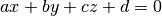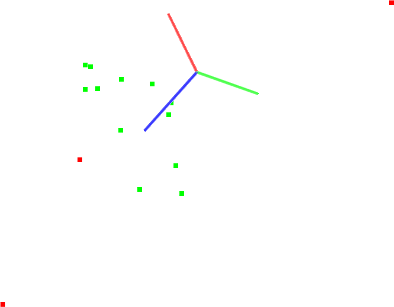# Plane model segmentation

In this tutorial we will learn how to do a simple plane segmentation of a set of points, that is to find all the points within a point cloud that support a plane model. This tutorial supports the Extracting indices from a PointCloud tutorial, presented in the filtering section.

# The code

First, create a file, let’s say, `planar_segmentation.cpp` in your favorite editor, and place the following inside it:

 ``` 1 2 3 4 5 6 7 8 9 10 11 12 13 14 15 16 17 18 19 20 21 22 23 24 25 26 27 28 29 30 31 32 33 34 35 36 37 38 39 40 41 42 43 44 45 46 47 48 49 50 51 52 53 54 55 56 57 58 59 60 61 62 63 64 65 66 67 68 69 70 71``` ```#include #include #include #include #include #include #include int main (int argc, char** argv) { pcl::PointCloud::Ptr cloud(new pcl::PointCloud); // Fill in the cloud data cloud->width = 15; cloud->height = 1; cloud->points.resize (cloud->width * cloud->height); // Generate the data for (auto& point: *cloud) { point.x = 1024 * rand () / (RAND_MAX + 1.0f); point.y = 1024 * rand () / (RAND_MAX + 1.0f); point.z = 1.0; } // Set a few outliers (*cloud).z = 2.0; (*cloud).z = -2.0; (*cloud).z = 4.0; std::cerr << "Point cloud data: " << cloud->size () << " points" << std::endl; for (const auto& point: *cloud) std::cerr << " " << point.x << " " << point.y << " " << point.z << std::endl; pcl::ModelCoefficients::Ptr coefficients (new pcl::ModelCoefficients); pcl::PointIndices::Ptr inliers (new pcl::PointIndices); // Create the segmentation object pcl::SACSegmentation seg; // Optional seg.setOptimizeCoefficients (true); // Mandatory seg.setModelType (pcl::SACMODEL_PLANE); seg.setMethodType (pcl::SAC_RANSAC); seg.setDistanceThreshold (0.01); seg.setInputCloud (cloud); seg.segment (*inliers, *coefficients); if (inliers->indices.size () == 0) { PCL_ERROR ("Could not estimate a planar model for the given dataset."); return (-1); } std::cerr << "Model coefficients: " << coefficients->values << " " << coefficients->values << " " << coefficients->values << " " << coefficients->values << std::endl; std::cerr << "Model inliers: " << inliers->indices.size () << std::endl; for (std::size_t i = 0; i < inliers->indices.size (); ++i) for (const auto& idx: inliers->indices) std::cerr << idx << " " << cloud->points[idx].x << " " << cloud->points[idx].y << " " << cloud->points[idx].z << std::endl; return (0); } ```

# The explanation

Now, let’s break down the code piece by piece.

Lines:

```#include <pcl/sample_consensus/method_types.h>
#include <pcl/sample_consensus/model_types.h>
#include <pcl/segmentation/sac_segmentation.h>
```

Important

Lines:

```  // Fill in the cloud data
cloud->width  = 15;
cloud->height = 1;
cloud->points.resize (cloud->width * cloud->height);

// Generate the data
for (auto& point: *cloud)
{
point.x = 1024 * rand () / (RAND_MAX + 1.0f);
point.y = 1024 * rand () / (RAND_MAX + 1.0f);
point.z = 1.0;
}

// Set a few outliers
(*cloud).z = 2.0;
(*cloud).z = -2.0;
(*cloud).z = 4.0;

std::cerr << "Point cloud data: " << cloud->size () << " points" << std::endl;
for (const auto& point: *cloud)
std::cerr << "    " << point.x << " "
<< point.y << " "
<< point.z << std::endl;
```

create the point cloud structure, fill in the respective values, and display the content on screen. Note that for the purpose of this tutorial, we manually added a few outliers in the data, by setting their z values different from 0.

Then, lines:

```  pcl::ModelCoefficients::Ptr coefficients (new pcl::ModelCoefficients);
pcl::PointIndices::Ptr inliers (new pcl::PointIndices);
// Create the segmentation object
pcl::SACSegmentation<pcl::PointXYZ> seg;
// Optional
seg.setOptimizeCoefficients (true);
// Mandatory
seg.setModelType (pcl::SACMODEL_PLANE);
seg.setMethodType (pcl::SAC_RANSAC);
seg.setDistanceThreshold (0.01);

seg.setInputCloud (cloud);
seg.segment (*inliers, *coefficients);
```

create the :pcl:`SACSegmentation <pcl::SACSegmentation>` object and set the model and method type. This is also where we specify the “distance threshold”, which determines how close a point must be to the model in order to be considered an inlier. In this tutorial, we will use the RANSAC method (pcl::SAC_RANSAC) as the robust estimator of choice. Our decision is motivated by RANSAC’s simplicity (other robust estimators use it as a base and add additional, more complicated concepts). For more information about RANSAC, check its Wikipedia page.

Finally:

```  std::cerr << "Model coefficients: " << coefficients->values << " "
<< coefficients->values << " "
<< coefficients->values << " "
<< coefficients->values << std::endl;
```

are used to show the contents of the inlier set, together with the estimated plane parameters (inform).

# Compiling and running the program

 ``` 1 2 3 4 5 6 7 8 9 10 11 12``` ```cmake_minimum_required(VERSION 2.8 FATAL_ERROR) project(planar_segmentation) find_package(PCL 1.2 REQUIRED) include_directories(\${PCL_INCLUDE_DIRS}) link_directories(\${PCL_LIBRARY_DIRS}) add_definitions(\${PCL_DEFINITIONS}) add_executable (planar_segmentation planar_segmentation.cpp) target_link_libraries (planar_segmentation \${PCL_LIBRARIES}) ```

After you have made the executable, you can run it. Simply do:

```\$ ./planar_segmentation
```

You will see something similar to:

```Point cloud data: 15 points
0.352222 -0.151883 2
-0.106395 -0.397406 1
-0.473106 0.292602 1
-0.731898 0.667105 -2
0.441304 -0.734766 1
0.854581 -0.0361733 1
-0.4607 -0.277468 4
-0.916762 0.183749 1
0.968809 0.512055 1
-0.998983 -0.463871 1
0.691785 0.716053 1
0.525135 -0.523004 1
0.439387 0.56706 1
0.905417 -0.579787 1
0.898706 -0.504929 1
[pcl::SACSegmentation::initSAC] Setting the maximum number of iterations to 50
Model coefficients: 0 0 1 -1
Model inliers: 12
1    -0.106395 -0.397406 1
2    -0.473106 0.292602 1
4    0.441304 -0.734766 1
5    0.854581 -0.0361733 1
7    -0.916762 0.183749 1
8    0.968809 0.512055 1
9    -0.998983 -0.463871 1
10    0.691785 0.716053 1
11    0.525135 -0.523004 1
12    0.439387 0.56706 1
13    0.905417 -0.579787 1
14    0.898706 -0.504929 1
```

A graphical display of the segmentation process is shown below.Note that the coordinate axes are represented as red (x), green (y), and blue (z). The points are represented with red as the outliers, and green as the inliers of the plane model found.